C# Tutorial

# How to use C# while loop

The C# while loop continually executes a block of statements until a specified expression evaluates to false . The expression is evaluated each time the loop is encountered and the evaluation result is true, the loop body statements are executed.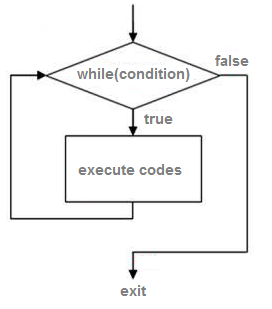Syntax
while(condition) { statement(s); }

Like if statement the while statement evaluates the expression, which must return a boolean value . If the expression evaluates to true, the while statement executes the statement(s) in the while block . The while statement continues testing the expression and executing its block until the expression evaluates to false.

### while loop example

using System; namespace ConsoleApplication1 { class Program { static void Main(string[] args) { int count = 1; while (count <= 4) { Console.WriteLine("The value of i is : " + count); count = count + 1; } Console.ReadKey(); } } }
output
The value of i is : 1 The value of i is : 2 The value of i is : 3 The value of i is : 4

The while loop executes a statement or a block of statements until a specified expression evaluates to false . The above C# while loop example shows the loop will execute the code block 4 times.

## How to terminate execution of while loop

A while loop can be terminated when a break , goto , return , or throw statement transfers control outside the loop. To pass control to the next iteration without exiting the loop, use the continue statement.

### break statement inside while loop

The break statement provides you with the opportunity to exit out of a while loop when an external condition is triggered. You will put the break statement within the block of code within your loop statement, normally after a conditional if statement. Statements in the loop after the break statement do not execute.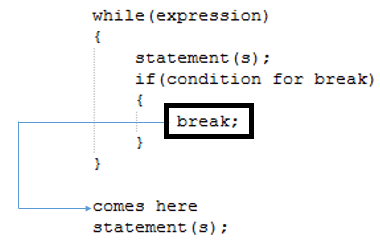The following program put a condition that when count is greater than 2 then exit from while loop.

if (count > 2) break;

When count greater than 2 the program will exit from while loop and goto next statement.

int count = 1; while (count <= 4) { Console.WriteLine("The value of i is : " + count); count = count + 1; if (count > 2) break; }
output
The value of i is : 1 The value of i is : 2

### continue statement inside while loop

When a continue statement is encountered inside a while loop, control forces to the beginning of the loop for next iteration , skipping the execution of statements inside the body of loop for the current iteration.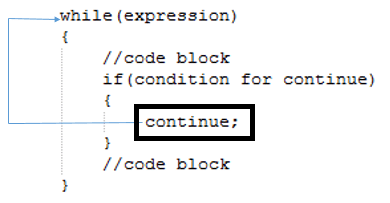int count = 1; while (count < 10) { count = count + 1; if (count < 5) continue; Console.WriteLine("The value of i is : " + count); }
example
The value of i is : 5 The value of i is : 6 The value of i is : 7 The value of i is : 8 The value of i is : 9 The value of i is : 10

## Break Vs Continue

1. break - looping is broken and stops.
2. continue - loop continues to execute with the next iteration.

### goto statement inside while loop

This goto keyword transfers control to a named label

int count = 1; while (count < 10) { count = count + 1; if (count >5) goto ReadKey; Console.WriteLine("The value of i is : " + count); } Console.WriteLine("Loop End here"); ReadKey: Console.WriteLine("Jump to here.....");
output
The value of i is : 2 The value of i is : 3 The value of i is : 4 The value of i is : 5 Jump to here.....

### return statement inside while loop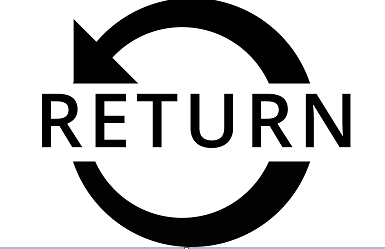You can return statement, but not recommended because it automatically exits from the current method of execution. Because, it is not useful when other statements after the loop are mandatory.

## Multiple condition in while loop

You can use multiple conditions in while loop. You can put multiple conditions using "& &" (AND), "" (OR) in while loop. Both & & and is "short-circuiting" operators, which means that if the answer is known from the left operand , the right operand is not evaluated.

## Nested while loop

A loop within another loop is called nested loop.while(condition) { while(condition) { statement(s); } statement(s); }
example
int outer = 0; while (outer < 2) { Console.WriteLine("Value of outer : " + outer); int inner = 0; outer++; while (inner < 3) { Console.WriteLine("Value of inner : " + inner); inner++; } }
output
Value of outer : 0 Value of inner : 0 Value of inner : 1 Value of inner : 2 Value of outer : 1 Value of inner : 0 Value of inner : 1 Value of inner : 2

## C# while(true) statement

An empty while-loop with this condition is by definition an infinite loop . You can implement an infinite loop using the while statement as follows:

while (true){ // statements }

## Empty statement

An empty statement is used when you no need to perform an operation where a statement is required. It simply transfers control to the end point of the statement.

using System; namespace ConsoleApplication1 { class Program { static int x; static void Main(string[] args) { // Use an empty statement as the body of the while-loop. while (foo(x++)) ; Console.ReadKey(); } static bool foo(int tX) { Console.WriteLine("Number : " + tX); return tX < 5; } } }
output
Number : 0 Number : 1 Number : 2 Number : 3 Number : 4 Number : 5

## Implicitly convert type error

When you run the following program you will get Implicitly convert type error .

class Program { static void Main() { int num = 1; while (num) { } } }

In C# while loop , you have to provide a condition(bool) (while(true)) so that the loop continues until the condition is met. You have instead provided an int instead of a condition(bool) , so while(num) is giving you an error. Just change it to while(num!=0) and that should give you a fix.

## C# while loop Vs. For loop

Internally, both loops (while and for) compile to the same machine code . The existence of two ways to repeat execution of code stems from two different use cases for each of them.

1. For loop knows in advance how many times it will loop, whereas a while loop doesn't know.
2. For loop has an initialization step whereas a while loop doesn't.
3. For loop uses a "step value" or increment/decrement step, whereas a while loop doesn't.
example
while(document.nextLine()) document.doStuff();

The while loop iterates through the lines of a document, and processing each line. It is not known in advance how many lines are there. This could not be done easily in a for loop, where you need to know in advance when you are going to stop.

for (int i = 0; i < 100; i++) { Console.WriteLine(i); }

Above is an example of a C# for loop looping from 0 to 99 and printing out the numbers. Notice how the loop is initialized and incremented as part of the for loop structure.

## C# while loop Vs. do..while loop

The while loop will check the condition first before executing the content.

while(test-condition) { statements; increment/decrement; }

The do while loop executes the content of the loop once before checking the condition of the while.

do { statements; increment/decrement; }while(test-condition);

## C# while loop programming exercises

1. Write a program to display first 10 odd numbers.
int i = 1; while (i <= 10) { Console.Write("{0} ", 2 * i - 1); i++; }
output
1 3 5 7 9 11 13 15 17 19

## Nested while loop examples

1. Write a program in to display the pattern like right angle triangle.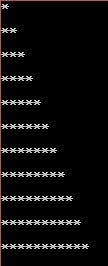int rows = 10; int i = 0; int j = 0; while (i<= rows) { j = 0; while (j <= i) { Console.Write("*"); j++; } Console.WriteLine("\n"); i++; }
1. Write a program to make such a pattern like a pyramid.int i, j, spc, rows, k; rows = 10; spc = rows + 4 - 1; i = 1; while ( i <= rows) { k = spc; while (k >= 1) { Console.Write(" "); k--; } for (j = 1; j <= i; j++) Console.Write("* "); Console.Write("\n"); spc--; i++; }
1. Write a program to display the pattern like a diamond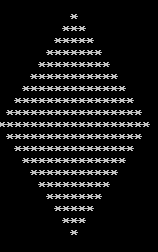int i, j, r; r = 10; i = 0; while (i <= r) { j=1; while (j <= r - i) { Console.Write(" "); j++; } j=1; while (j <= 2 * i - 1) { Console.Write("*"); j++; } Console.Write("\n"); i++; } i = r - 1; while (i >= 1) { j = 1; while ( j <= r - i) { Console.Write(" "); j++; } j = 1; while ( j <= 2 * i - 1) { Console.Write("*"); j++; } Console.Write("\n"); i--; }